JEE  >  Test: Refraction by Spherical Surfaces

# Test: Refraction by Spherical Surfaces

Test Description

## 5 Questions MCQ Test Physics Class 12 | Test: Refraction by Spherical Surfaces

Test: Refraction by Spherical Surfaces for JEE 2023 is part of Physics Class 12 preparation. The Test: Refraction by Spherical Surfaces questions and answers have been prepared according to the JEE exam syllabus.The Test: Refraction by Spherical Surfaces MCQs are made for JEE 2023 Exam. Find important definitions, questions, notes, meanings, examples, exercises, MCQs and online tests for Test: Refraction by Spherical Surfaces below.
Solutions of Test: Refraction by Spherical Surfaces questions in English are available as part of our Physics Class 12 for JEE & Test: Refraction by Spherical Surfaces solutions in Hindi for Physics Class 12 course. Download more important topics, notes, lectures and mock test series for JEE Exam by signing up for free. Attempt Test: Refraction by Spherical Surfaces | 5 questions in 10 minutes | Mock test for JEE preparation | Free important questions MCQ to study Physics Class 12 for JEE Exam | Download free PDF with solutions
 1 Crore+ students have signed up on EduRev. Have you?
Test: Refraction by Spherical Surfaces - Question 1

### A ray of light going from denser to rarer medium suffers refraction at a concave surface. Which of the following relations is correct?

Detailed Solution for Test: Refraction by Spherical Surfaces - Question 1

Solution :

The correct option is Option A.

Laws of refraction;-

The incident ray,the refracted ray and the normal to the refracting surface at the point of incidence lie in the same plane.

For a given pair of media and for a given colour of light the ration between the sine of angle of incidence to the sine of refraction is a constant.This constant is known as refractive index of the second medium with respect to the first medium.

When a ray of light passes through a glass slab, ∠i,∠r and the normal all lie in the same plane.

When a ray of light passes from one medium to another, here from air to glass or glass to air, the ratio sini / sinr = constant.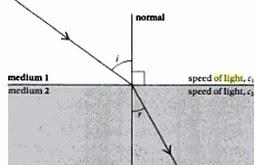Test: Refraction by Spherical Surfaces - Question 2

### For a plane surface, the formula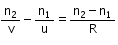becomes

Detailed Solution for Test: Refraction by Spherical Surfaces - Question 2

For plane mirror R=infinity
so. (n2-n1)/R=0
therefore, n2/V=n1/U

Test: Refraction by Spherical Surfaces - Question 3

### Consider a curved surface between two different media with refractive indices n1 = 1 and n2 = 2. The relation between radius of curvature, image distance and object distance is given by

Detailed Solution for Test: Refraction by Spherical Surfaces - Question 3

The relation between radius of curvature, image distance and object distance is given by
n2/v-n1/u=n2-n1
un2-vn2/vu=n2-n1/R
R=vu(n2-n2)/un2-vn2
=uv(2)/u(2)-v(1)
=[uv/2u-v)

Test: Refraction by Spherical Surfaces - Question 4

In the adjoining figure, SS is a spherical surface separating two media of refractive indices n1 and n2 where n1 > n2. C is the centre of curvature of the spherical surface. An observer, keeping his eye beyond C in the medium of refractive index n2 views the refracted image of an object AB placed as shown in the medium of refractive index n1. The image will be: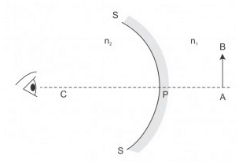Detailed Solution for Test: Refraction by Spherical Surfaces - Question 4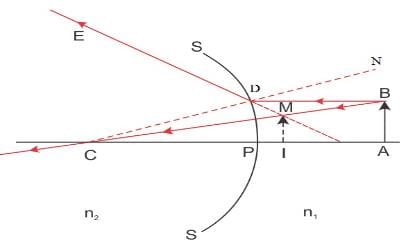The image will be virtual, upright and diminished.

Test: Refraction by Spherical Surfaces - Question 5

A thick plano convex lens made of crown glass (refractive index 1.5) has a thickness of 3cm at its centre. The radius of curvature of its curved face is 5cm. An ink mark made at the centre of its plane face, when viewed normally through the curved face, appears to be at a distance ‘x’ from the curved face. Then, x is equal to: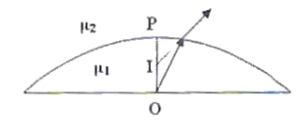Detailed Solution for Test: Refraction by Spherical Surfaces - Question 5

A thick Plano convex lens made of crown glass (refractive index 1.5) has a thickness of 3cm at its centre. The radius of curvature of its curved face is 5cm. An ink mark made at the centre of its plane face, when viewed normally through the curved face, appears to be at a distance ‘x’ from the curved face, and we have to determine the value of ' x'
According to the picture, the ray of light gets refracted at the interface between the air and the lens from the object 'p' and 'I' is the refracted image of 'p'
object distance 'u'= BO
Image distance 'v'/'x' = BI
we know
n2/v - n1/u = (n2- n1) / R
or 1/v - 1.5/(-3 )= (1-1.5)/ (-5) [where n2= 1, n1= 1.5 ,u= -3 ,R= -5]
so, 1/v = -6/15
or, v = -2.5
so, x is equal to 2.5 cm

## Physics Class 12

157 videos|452 docs|213 tests
 Use Code STAYHOME200 and get INR 200 additional OFF Use Coupon Code
Information about Test: Refraction by Spherical Surfaces Page
In this test you can find the Exam questions for Test: Refraction by Spherical Surfaces solved & explained in the simplest way possible. Besides giving Questions and answers for Test: Refraction by Spherical Surfaces, EduRev gives you an ample number of Online tests for practice

## Physics Class 12

157 videos|452 docs|213 tests